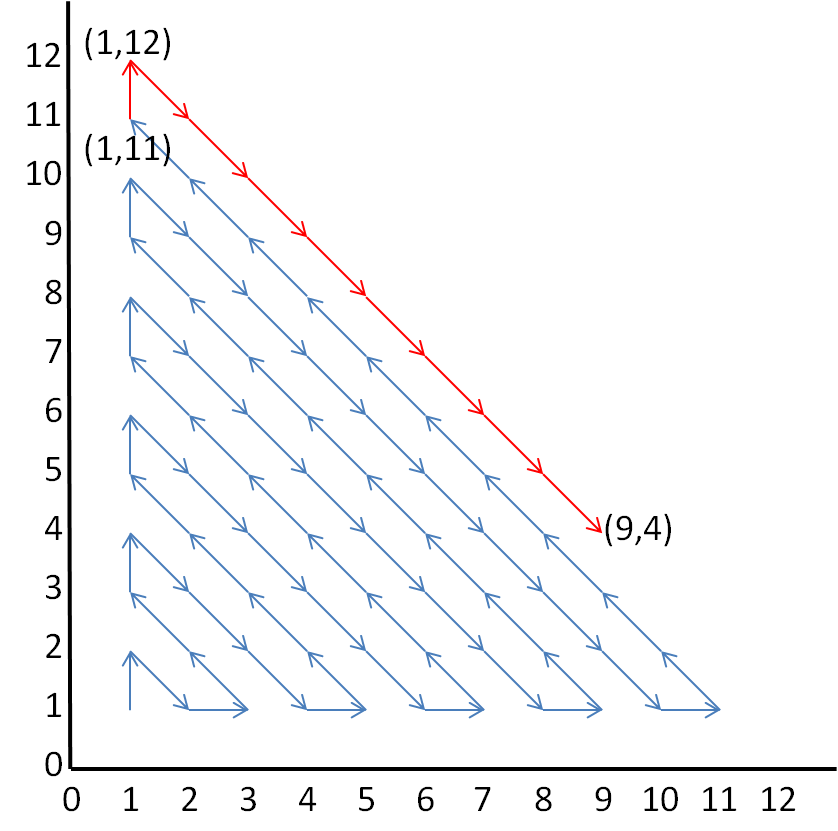#### You may also like### Arithmagons Poster

Arithmagons Poster### Squares in Rectangles Poster

Squares In Rectangles Poster### Isosceles Triangles Poster

Isosceles Triangles Poster - February 2005

# Route to Infinity Poster

##### Age 11 to 14Challenge Level

The route will visit (18,17), and the next number after that will be (19,16). The route will visit 74 points before it reaches (9,4).

Why will it visit (19,16) after (18,17)?

Method 1: 'equal' coordinates
The route goes upwards through the points with coordinates that are equal (eg. (18,18)) and downwards through the points with coordinates that are adjacent (eg. (18,17)). Therefore the next coordinate is (19,16).

Method 2: ends of lines
Looking at the diagram you can tell that if the x coordinate of the bottom right point on a line is even, the arrows in the line go down and right, otherwise, they go up and left. The point (18,17) is on the line with bottom right point (18+17-1,1) = (34,1). Because 34 is even, the arrows go down and right, so the next point is (19,16).

Method 2 can also be written algebraically:
The numbers all lie on lines with equations $x+y=n,$ where $n$ is some integer. If $n$ is odd, then the line begins at $(1, n-1).$ These arrows point towards the bottom right. If $n$ is even, the line begins at coordinate $(n-1, 1)$ and the direction of the line is towards the top left. Notice that $18+17=35,$ so the coordinate $(18, 17)$ is on the line $x+y=35.$

How do you know it will visit 74 points before it reaches (9,4)?

The number of points visited follows a pattern.
Number of points visited up to (1,1): 1
Number of points visited up to (2,1): 3
Number of points visited up to (1,3): 6
Number of points visited up to (4,1): 10

These are the triangle numbers: 1+ 2 = 3, 1 + 2 + 3 = 6, 1 + 2 + 2 + 4 = 10, etc.
Therefore the number of points visited up to (1,11) is 66.

9+4 = 13 is on the same line as (1,12) and (12,1), which is a line pointing towards the bottom right.

So after (1,11), the route will visit (1,12), (2,11), (3,10), ... (8,5) before (9,4), which is 8 more points.

66 + 8 = 74

This is shown in the diagram below.